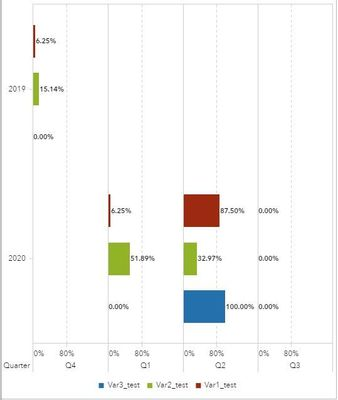## How to calculate a percent of total of quarter?

Hello! I am using SAS VA 7.5. I would like to calculate percent of total for quarter and display results in a bar graph to see change over time. Below is an example of how my data are structured and an example of the desired graph. The category=year and lattice columns=quarter.

 Q Var1 Q1 0 Q1 1 Q1 0 Q1 0 Q2 0 Q2 1 Q2 1 Q2 0 Q3 0 Q3 1 Q3 1 Q3 1So I would like to visualize Var1 going from 25% to 50% to 75% over Qs 1-3. I experimented with right-clicking the variable, creating new calculation, and percent of total but this, of course, used all data and didn't segment by quarter. I have also tried creating a new calculated variable from the data pane and creating an aggregated measure but it was incorrect.

I'm also curious what dates VA uses for it's preset quarters in aggregations. I need to use non-standard dates (e.g. Q1=Oct-Dec) which should factor into the calculation.

Any suggestions are appreciated, thank you!

1 ACCEPTED SOLUTION

Accepted Solutions

## Re: How to calculate a percent of total of quarter?

Try creating an aggregated measure that looks like the following:

sum(_bygroup_(Var0))/sum(_ForAll_(Var0))

2 REPLIES 2

## Re: How to calculate a percent of total of quarter?

Try creating an aggregated measure that looks like the following:

sum(_bygroup_(Var0))/sum(_ForAll_(Var0))

## Re: How to calculate a percent of total of quarter?

That worked perfectly, thank you!
Discussion stats
• 2 replies
• 727 views
• 0 likes
• 2 in conversation